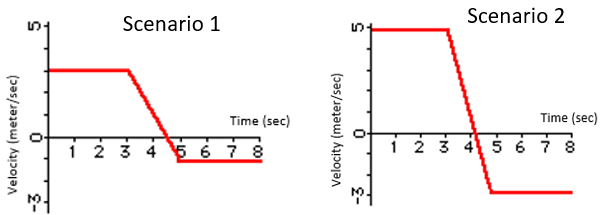## Velocity-Time Graph

Consider the two velocity vs time graphs below.
1. Which scenario has the greater velocity change?
2. Which scenario has the greater acceleration?
3. Which scenario has the greater momentum change?
4. Which scenario has the greater impulse?Hint
$$Force=mass\cdot acceleration=mass\cdot \frac{\Delta velocity}{\Delta time}$$$Hint 2 $$Impulse=Change\:in\:momentum$$$
Looking at the two graphs, both scenarios are experiencing a velocity change from the three to five second mark (x-axis). Therefore, if we evaluate the change in the y-axis direction (velocity) for this time range, we can determine the greater velocity change.
$$v_1=3-(-1)=4\:m/s$$$$$v_2=5-(-3)=8\:m/s$$$
(1.) Scenario 2 has the larger velocity change.

Acceleration is defined as:
$$acceleration=\frac{\Delta velocity}{\Delta time}$$$$$a_1=\frac{4}{2}=2\:m/s^2$$$
$$a_2=\frac{8}{2}=4\:m/s^2$$$(2.) Scenario 2 has the larger acceleration. Impulse is defined as $$force\cdot time$$ , while momentum is defined as $$mass\cdot velocity$$ , and the change in momentum is $$mass\cdot \Delta velocity$$ . Therefore, if we multiple both sides of Newton' second law ( $$F=ma$$ ) equation by $$\Delta t$$ : $$Force \cdot \Delta time=mass\cdot \Delta velocity$$$
$$Impulse=Change\:in\:momentum$$\$
(3.) Scenario 2 has the larger momentum change. Since mass is constant, the velocity change will dictate the greater momentum change.
(4.) Scenario 2 has the larger impulse. Impulse is momentum change, and the momentum change is greatest in scenario 2.
(1.) Scenario 2 has the larger velocity change.
(2.) Scenario 2 has the larger acceleration.
(3.) Scenario 2 has the larger momentum change.
(4.) Scenario 2 has the larger impulse.